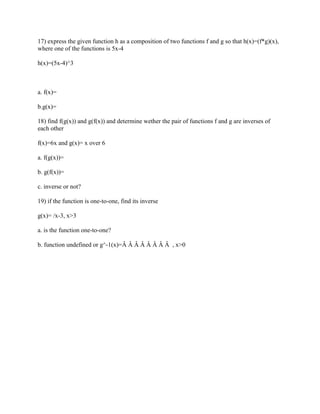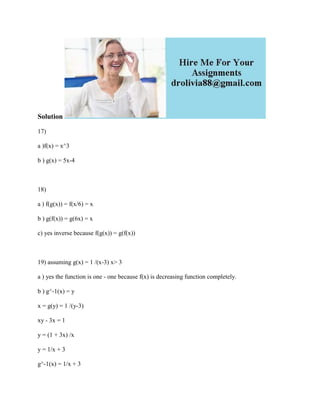Successfully reported this slideshow.

# 17) express the given function h as a composition of two functions f a.docx×

# 17) express the given function h as a composition of two functions f a.docx

17) express the given function h as a composition of two functions f and g so that h(x)=(f*g)(x), where one of the functions is 5x-4
h(x)=(5x-4)^3

a. f(x)=
b.g(x)=
18) find f(g(x)) and g(f(x)) and determine wether the pair of functions f and g are inverses of each other
f(x)=6x and g(x)= x over 6
a. f(g(x))=
b. g(f(x))=
c. inverse or not?
19) if the function is one-to-one, find its inverse
g(x)= /x-3, x>3
a. is the function one-to-one?
b. function undefined or g^-1(x)=Â Â Â Â Â Â Â Â  , x>0

Solution
17)
a )f(x) = x^3
b ) g(x) = 5x-4

18)
a ) f(g(x)) = f(x/6) = x
b ) g(f(x)) = g(6x) = x
c) yes inverse because f(g(x)) = g(f(x))

19) assuming g(x) = 1 /(x-3) x> 3
a ) yes the function is one - one because f(x) is decreasing function completely.
b ) g^-1(x) = y
x = g(y) = 1 /(y-3)
xy - 3x = 1
y = (1 + 3x) /x
y = 1/x + 3
g^-1(x) = 1/x + 3
.

17) express the given function h as a composition of two functions f and g so that h(x)=(f*g)(x), where one of the functions is 5x-4
h(x)=(5x-4)^3

a. f(x)=
b.g(x)=
18) find f(g(x)) and g(f(x)) and determine wether the pair of functions f and g are inverses of each other
f(x)=6x and g(x)= x over 6
a. f(g(x))=
b. g(f(x))=
c. inverse or not?
19) if the function is one-to-one, find its inverse
g(x)= /x-3, x>3
a. is the function one-to-one?
b. function undefined or g^-1(x)=Â Â Â Â Â Â Â Â  , x>0

Solution
17)
a )f(x) = x^3
b ) g(x) = 5x-4

18)
a ) f(g(x)) = f(x/6) = x
b ) g(f(x)) = g(6x) = x
c) yes inverse because f(g(x)) = g(f(x))

19) assuming g(x) = 1 /(x-3) x> 3
a ) yes the function is one - one because f(x) is decreasing function completely.
b ) g^-1(x) = y
x = g(y) = 1 /(y-3)
xy - 3x = 1
y = (1 + 3x) /x
y = 1/x + 3
g^-1(x) = 1/x + 3
.